A dataset for question answering and reading comprehension from a set of Wikipedia articles

The Stanford Question Answering Dataset (SQuAD) consists of questions posed by crowd workers on a set of Wikipedia articles, where the answer to every question is a segment of text, or span, from the corresponding reading passage.

Modifications from the original dataset: Data marked "Training" in the ValidationRole field corresponds to the Training Set v1.1 subset of the original dataset. Data marked "Validation" in the ValidationRole field corresponds to the Dev Set v1.1 subset of the original dataset. The original dataset is 0-indexed, in order be accurate in the Wolfram Language, 1 was added to the value of "AnswerPosition", as the Wolfram Language is 1-indexed.

Examples

Basic Examples

Retrieve the resource:

 In:=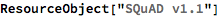Out=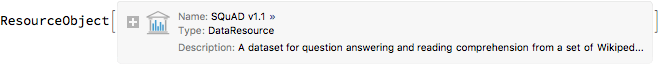Retrieve a sample of the dataset:

 In:=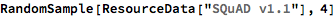Out=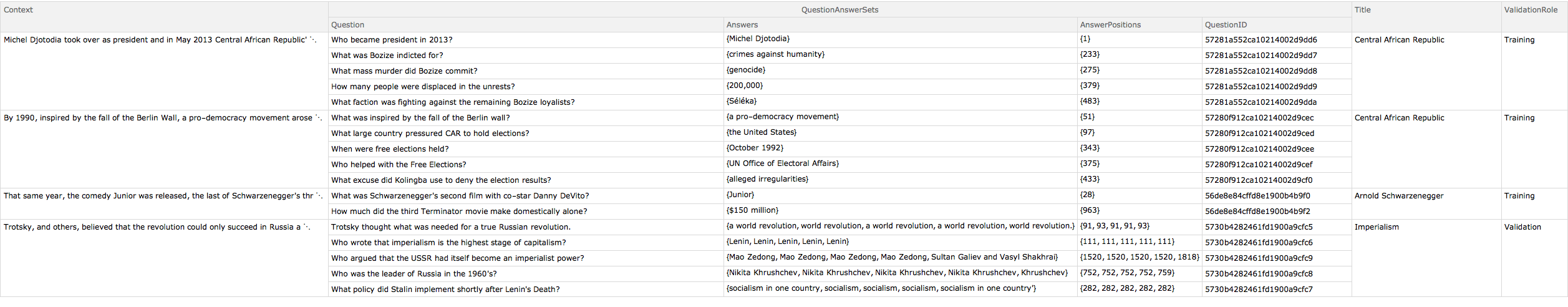Working with Training and Validation data:

Obtain the first example from the training dataset:

 In:=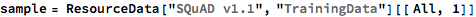Out=View the question and answer associated with the passage:

 In:=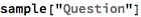Out=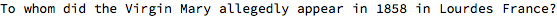In:=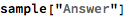Out=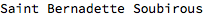"AnswerPosition" shows where the first character of the answer is located in the passage. The position of the last character can be computed:

 In:=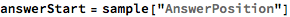Out=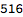In:=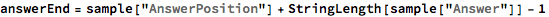Out=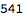Test that the span between the starting position and the ending position is equivalent to the answer:

 In:=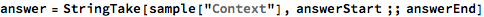Out=In:=Out=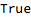In:=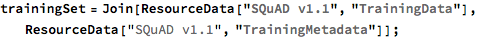There is a one-to-one correspondence between the data and the metadata, therefore all lists will have the same length:

 In:=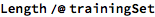Out=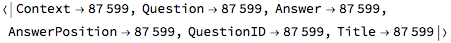View an example of the joined data:

 In:=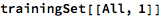Out=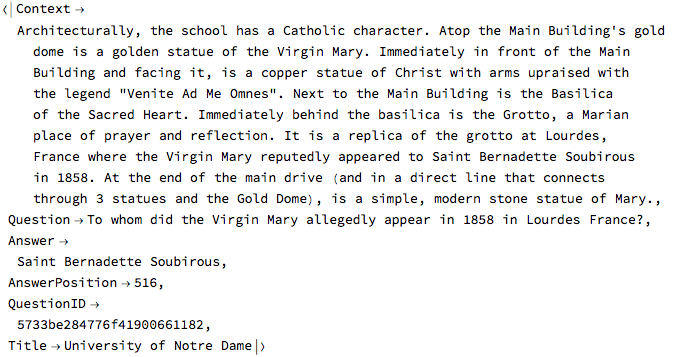Aggregation

Set the types of questions:

 In:=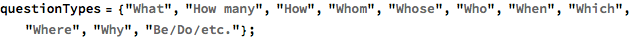Define the patterns corresponding to these questions:

 In:=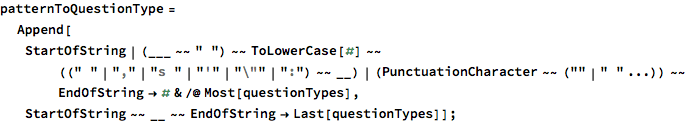Classify the questions from the SQuAD dataset:

 In:=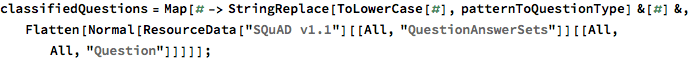Print the statistics about the question types

 In:=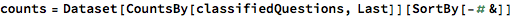Out=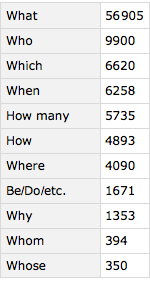In:=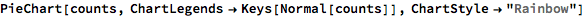Out=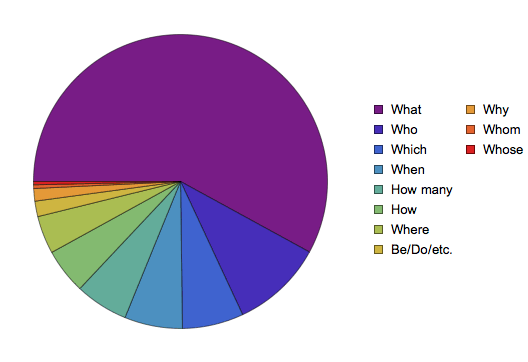Display some examples for each type of question:

 In:=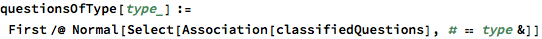In:=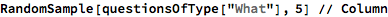Out=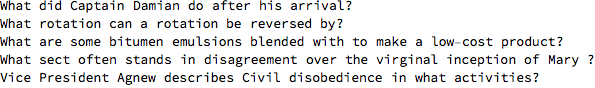In:=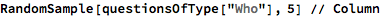Out=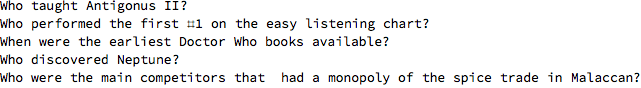In:=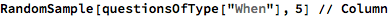Out=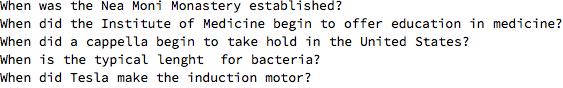In:=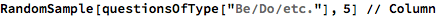Out=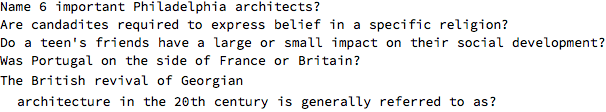Dataset size

 In:=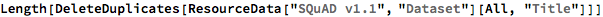Out=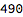Display the number of Wikipedia paragraphs:

 In:=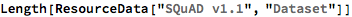Out=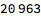Display the number of question/answer pairs in the training and validation datasets:

 In:=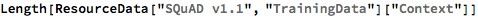Out=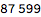In:=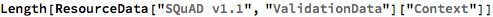Out=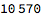Wolfram Research, "SQuAD v1.1" from the Wolfram Data Repository (2018)